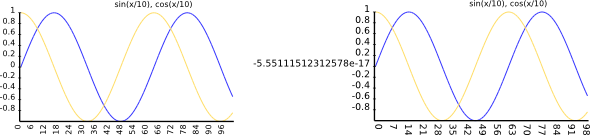# Rats and other pets

If you follow perlmonks (or just about any other programming forum) you'll notice recurring questions related to floating point arithmetics not being accurate. A typical example would be

```my \$a =  1 / 10;
my \$b = 1;
for ( 1..10 ) {
\$b -= \$a;
}
if ( \$b == 0 ) {
print "yes\n";
} else {
print "no\n";
}

printf "%.20f\n", \$b;
```

When you run this with perl5 the output is

```no
0.00000000000000013878
```

And the reason is that `1/10` is an infinite binary fraction, and perl uses floating points internally with only a finite number of bits for the mantissa. So it subtracts ten times not exactly one tenth, but a number close to that, and in the end the result is not exactly zero, but of the order of magnitude of the machine precision.

However when you run the very same program with Perl 6, you get

```yes
0.00000000000000000000
```

Why? Introspection helps:

```\$ perl6 -e 'say (1/10).WHAT'
Rat()
\$ perl6 -e 'say (1/10).perl'
1/10
```

So `1/10` produces not a floating point number, but a `Rat` object. Rat is short for Rational, and it's a fraction that stores the numerator and denominator as an integer each, allowing exact arithmetics without floating point errors.

Here is a real world example where this makes a difference:These two plots are both made with SVG::Plot; the right hand side uses floats, the left hand side uses Rats. With floats the zero axis label is not exactly zero, but a small number close to zero. Instead of making a special case for the zero label, or introducing complicated rounding rules, I switched to Rats - which just implied changing a `5.0` (Num) to `5` (Int).

(Back in the days when I used pugs for Perl 6 programming I found the extensive use of rationals quite annoying, because when I wanted to calculate `1/7` it would answer me with `1/7` - thank you, I knew that already. The current specification says that rationals should delegate string representation to Num (aka floating point numbers), so `say 1/7` produces `0.142857142857143`. This is very handy, but means that conversion to Str and back to a number loses precision. Works for me.)

(frettled gets credit for suggesting the title of this blog post).